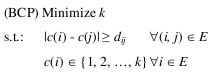# Bandwidth Coloring Problem

Martí, Gortázar and Duarte (2009)
Optsicom project, University of Valencia (Spain)

# Problem Description

The bandwidth coloring problem consists of assigning a color to each vertex of a graph, so that the absolute value of the difference between the colors of adjacent vertices is at least the value of the weight of the associated edge. This problem generalizes the classical vertex coloring problem.

Let G=(V,E) be a graph with a vertex set V (|V| = n) and an edge set E (|E|= m) with a strictly positive integer weight dij associated to each edge (i, j) ∈ E. A k-coloring c of G labels each vertex iV with an integer c(i) ∈ {1, 2, ..., k} (called color) in such a way that |c(i) - c(j)| ≥ dij for all (i, j) ∈ E. The bandwidth coloring problem (BCP) consists of finding a k coloring with the smallest value of k. In mathematical terms:The classical vertex coloring problem (VCP) is a particular case of the BCP in which dij=1 for all (i, j) ∈ E. The BCP is therefore NP-hard since it generalizes the VCP (Garey and Johnson 1979).

# State of the Art Methods

The most relevant approximated methods developed to solve this problem are:

• FCNS: Constructive method that performs forward checking to avoid forbidden colorings. Combines a local search with a constraint propagation algorithm. Prestwich (2002)
• Multistart: A multi-start method that first generates a sequence of nodes and then produces a solution of the BCP with a greedy algorithm. Then, it tries to improve this solution by reducing the number of colors used by one unit below the number of colors in the best solution known so far. Lim et al. (2005)
• DSATUR+T1: A combination of evolutionary and tabu search methodologies. Malaguti and Toth (2008)
• AMP: GRASP algorithm with an ejection chain improvement method that uses memory structures. Martí, Gortázar and Duarte (2009)

# Instances (BCPLIB)

The instance set GEOM contains the instances reported in most of the previous BCP papers. GEOM consists of 33 geometric graphs generated by Michael Trick. In these graphs, points are generated in a 10,000 by 10,000 grid and are connected by an edge if they are close enough together. Edge weights are inversely proportional to the distance between nodes. This set contains three types of graphs. The GEOMn instances are sparse, the GEOMna and GEOMnb instances are denser.

# Computational Experience

We performed a computational comparison of the state of the art methods. We have implemented the methods in Java SE 6 and the experiment was conducted on a Pentium 4 computer at 3 GHz with 2 GB of RAM. It can be downloaded in Excel.

# References (Best Methods)

• Garey, M.R. and D.S. Johnson (1979) Computers and Intractability. A guide to the theory of completeness, W. H. Freeman and Company, New York.
• Prestwich, S. (2002) "Constrained bandwidth multicoloration neighborhoods" Computational symposium on graph coloring and its generalizations, Ithaca, NY, 126-133.
• Lim, A., Y. Zhu, Q. Lou and B. Rodrigues (2005) "Heuristic Methods for Graph Coloring Problems," The 20th Annual ACM Symposium on Applied Computing (SAC 2005, Santa Fe, New Mexico) March 13-17.
• Malaguti, E. and P. Toth (2008) "An evolutionary approach for bandwidth multicoloring problems", European Journal of Operational Research 189, 638-651.
• Martí, R., F. Gortázar and A. Duarte (2009) "Heuristics for the Bandwidth Coloring Problem", International Journal of Metaheuristics, to be published.